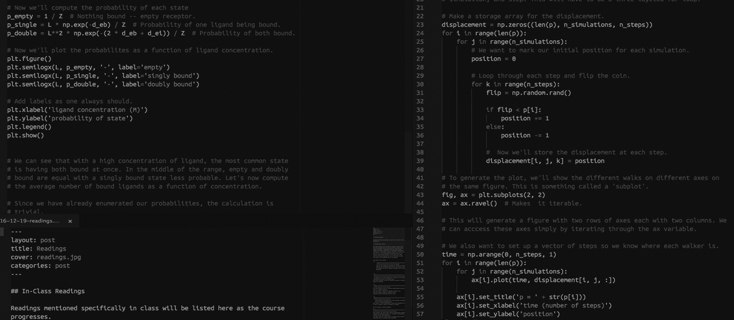# Code

## Exercises

The code we write in class will be added here as the course progresses.

• Integrating Cell Division by the Euler Method | Here, we get introduced to the MATLAB programming language and learn how to numerically solve the differential growth equation. pdf

• How the Fly Gets Its Neck | In this tutorial we go through the theory and analysis of the cephalic furrow positioning in the fly embryo. html | mlx

• Measuring Growth Rate Through Microscopy | We’ve all (probably) measured bacterial growth by recording the OD of growing cultures. In this script, we analyze a time-lapse microscopy data set by measuring the bacterial area as a function of time. | in-class code | data set

• Timing Transcription in Drosophila embryos | Using some of the image processing skill we learned while processing the growth movie, we measure the rate of transcription of the hunchback gene. html | mlx

• Nonlinear Regression | We performed a nonlinear regression of our LacI titration data to estimate the best fit value for the binding energy of a repressor to the DNA.

• Cytoskeleton length distribution | Data analysis of cytoskeleton images to obtain the length distribution and compare it with the theoretical predictions from the chemical master equation model. html | mlx

## Advance Matlab tutorial (introduction to Bayesian inference)

• Intro to Bayesian inference | First exposition to the concept of Bayesian parameter inference. [ html ] | [ mlx ] | [ raw data ]

• Intro to Markov Chain Monte Carlo sampling | Introduction to the powerful MCMC techniques widely used for Bayesian analysis. [ html ] | [ mlx ]

## Extra Tutorials

We have written several more in-depth tutorials on programming in the MATLAB programming language and have posted them below. More will be posted as the course progresses.

## External Resources

Below is a list of useful online resources for learning the some principles of programming and scientific data analysis using the Python language.